Question

Let X and Y be two random variables with joint probability mass function:

(?,?) = (??(3+?))/(18*3+30)??? ?=1,2,3 ??? ?=1,2

(?,?) = 0, Otherwise.

• Find P(X>Y)

and

Let X and Y be two random variables with joint probability mass function:

(?,?) = (??(4+?))/(18*4+30)??? ?=1,2,3 ??? ?=1,2

(?,?) = 0, Otherwise.

• Find P(Y=2/X=1)

1)P(X>Y)=P(X=2,Y=1)+P(X=3,Y=1)+P(X=3,Y=2)=2*1*(3+1)/84+3*1*(3+1)/84+3*2*(3+2)/84=0.595

2)P(Y=2|X=1)=P(X=1,Y=2)/P(X=1)=0.117647/0.1666667=0.706

#### Earn Coins

Coins can be redeemed for fabulous gifts.

Similar Homework Help Questions
• ### The joint probability mass function of random variables X and Y is given by if x1...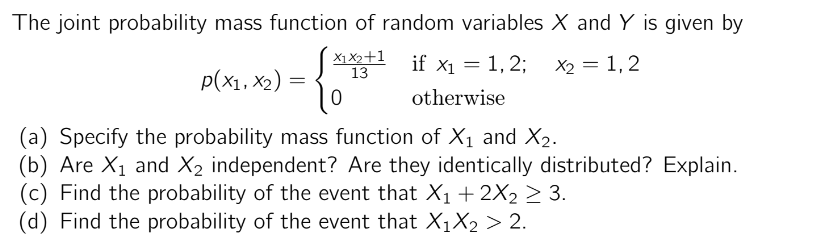The joint probability mass function of random variables X and Y is given by if x1 = 1,2; x2 = 1,2 p(x1, x2) = { otherwise (a) Specify the probability mass function of X1 and X2. (b) Are X1 and X2 independent? Are they identically distributed? Explain. (C) Find the probability of the event that X1 + 2X2 > 3. (d) Find the probability of the event that X1 X2 > 2.

• ### 3. Let the random variables X and Y have the joint probability density function 0 y...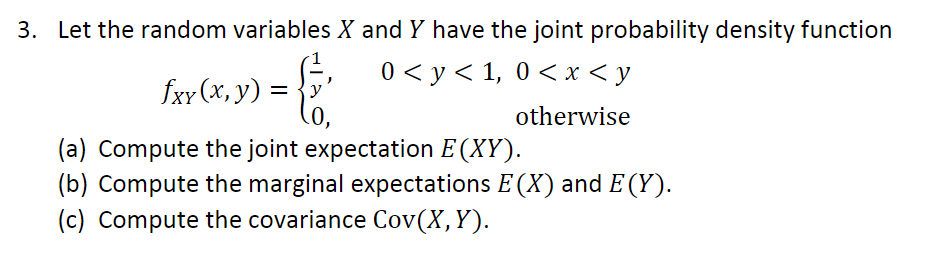3. Let the random variables X and Y have the joint probability density function 0 y 1, 0 x < y fxy(x, y)y otherwise (a) Compute the joint expectation E(XY) (b) Compute the marginal expectations E(X) and E (Y) (c) Compute the covariance Cov(X, Y)

• ### 3. Let the random variables X and Y have the joint probability density function fxr (x,...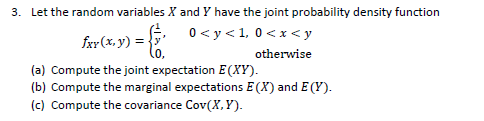3. Let the random variables X and Y have the joint probability density function fxr (x, y) = 0 <y<1, 0<xsy otherwise (a) Compute the joint expectation E(XY). (b) Compute the marginal expectations E(X) and E(Y). (c) Compute the covariance Cov(X,Y).

• ### . Let X and Y be the proportion of two random variables with joint probability density...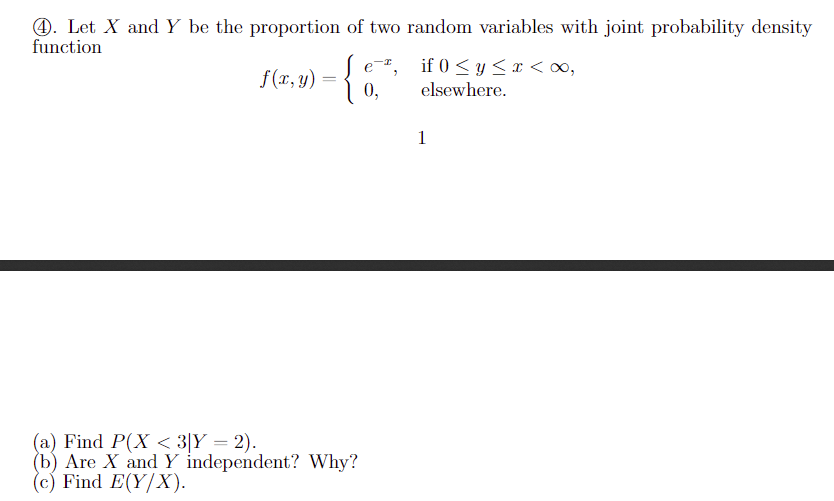. Let X and Y be the proportion of two random variables with joint probability density function f(r, y) e-*, 0, if, 0 < y < x < oo, elsewhere. a) Find P(Xc3.y-2). b) Are X and Y independent? Why? c) Find E(Y/X)

• ### Let X and Y be random variables with joint probability density function f(x, y) = {Cxe...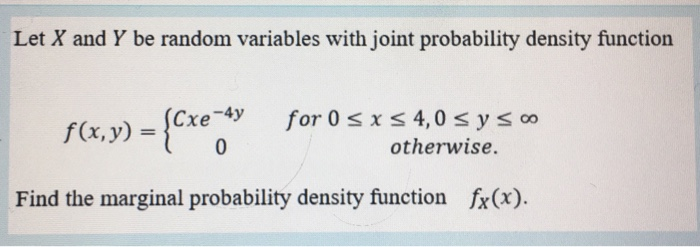Let X and Y be random variables with joint probability density function f(x, y) = {Cxe for 0 SXS 4,0 s y soo otherwise. Find the marginal probability density function fx(x).

• ### . Let X and Y be the proportion of two random variables with joint probability density...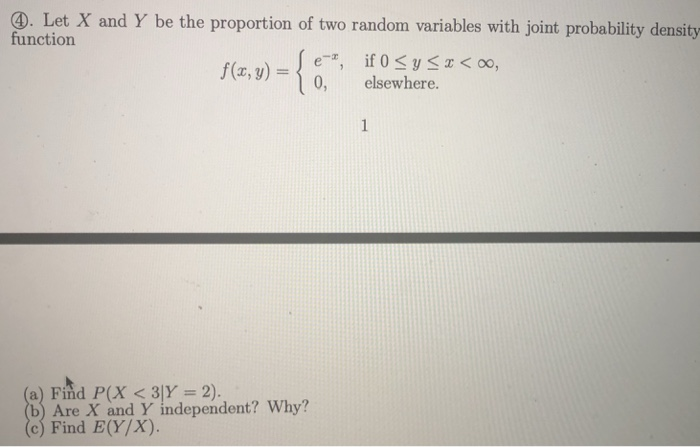. Let X and Y be the proportion of two random variables with joint probability density function f(x, y)o, elsewhere. (a) Find P(X < 3|Y= 2). (b) Are X and Y independent? Why? (c) Find E(Y/X)

• ### . Let X and Y be the proportion of two random variables with joint probability density.... Let X and Y be the proportion of two random variables with joint probability density function f(x, y)o, elsewhere. (a) Find P(X < 3|Y= 2). (b) Are X and Y independent? Why? (c) Find E(Y/X)

• ###  The joint probability mass function of two discrete random variables A and B is PAB(a,...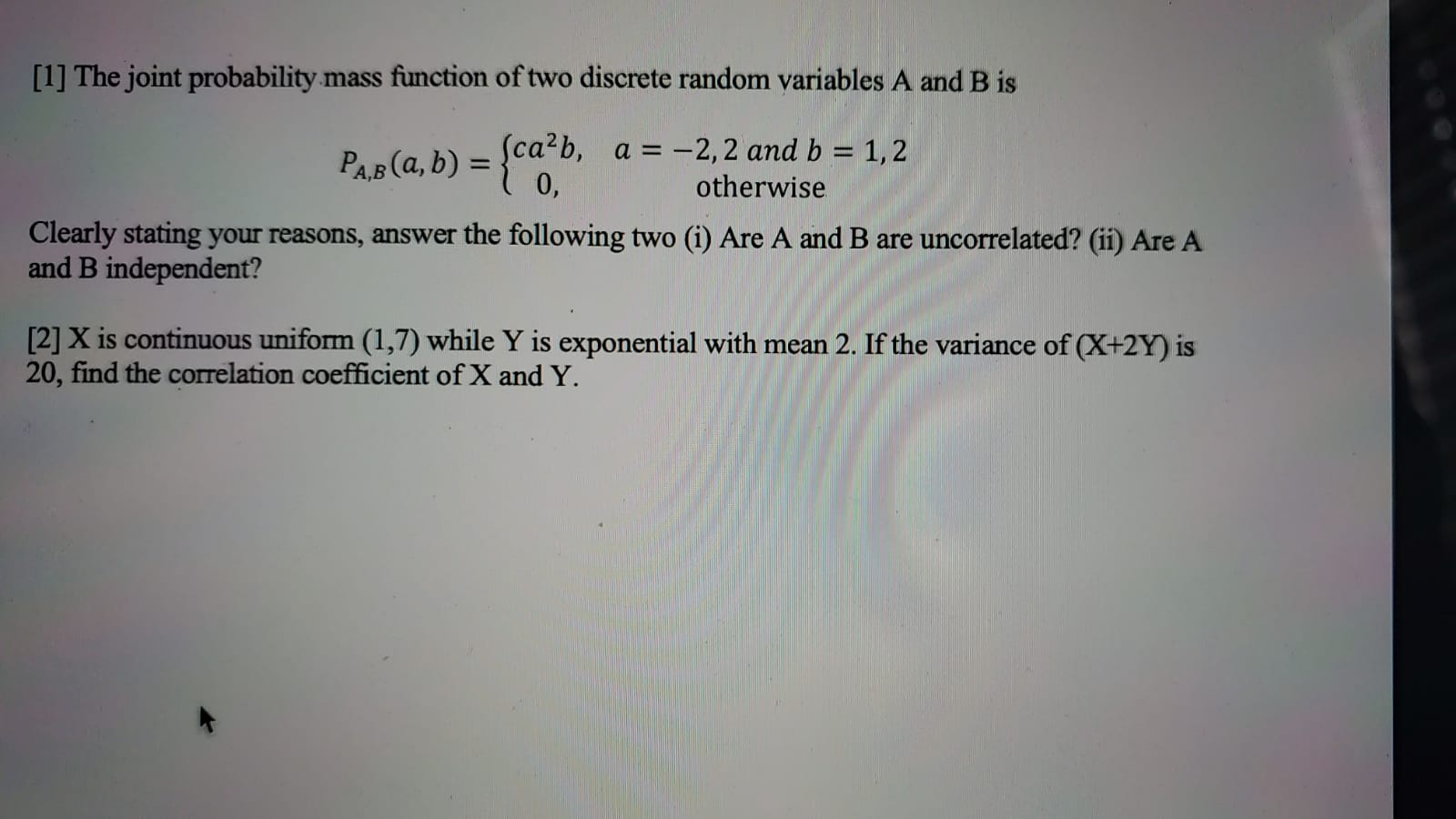The joint probability mass function of two discrete random variables A and B is PAB(a, b) = Sca²b, a = -2,2 and b = 1,2 0, otherwise Clearly stating your reasons, answer the following two (i) Are A and B are uncorrelated? (ii) Are A and B independent?  X is continuous uniform (1,7) while Y is exponential with mean 2. If the variance of (X+2Y) is 20, find the correlation coefficient of X and Y.

• ### 5. Random variables X and Y have joint probability mass function otherwise (a) Find the value...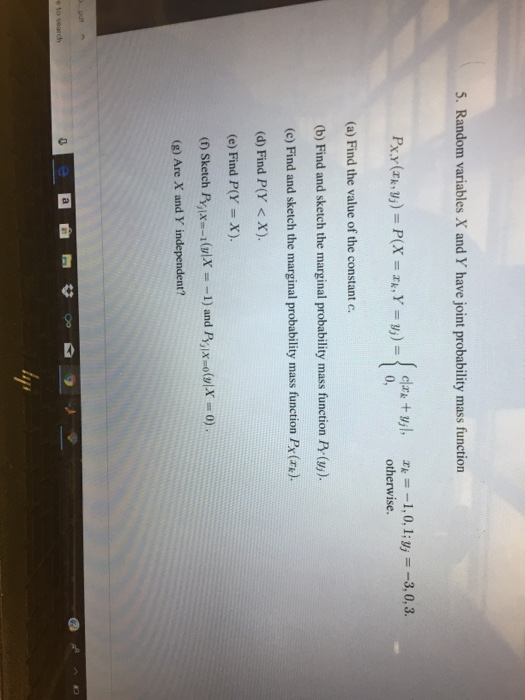5. Random variables X and Y have joint probability mass function otherwise (a) Find the value of the constant c. (b) Find and sketch the marginal probability mass function Py (u). (c) Find and sketch the marginal probability mass function Px (rk). (d) Find P(Y <X). (e) Find P(Y X) (g) Are X and Y independent? 2 内?

• ### Let the random variable X and Y have the joint probability density function. fxy(x,y) lo, 3....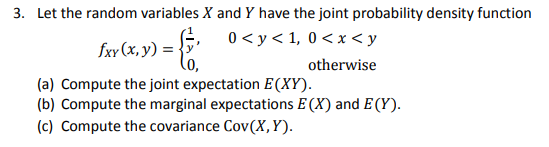Let the random variable X and Y have the joint probability density function. fxy(x,y) lo, 3. Let the random variables X and Y have the joint probability density function fxy(x, y) = 0<y<1, 0<x<y otherwise (a) Compute the joint expectation E(XY). (b) Compute the marginal expectations E(X) and E(Y). (c) Compute the covariance Cov(X,Y).